Mechanical Engineering - Mechanics                                 Home : www.sharetechnote.com Mechanics : Curvilinear 2D   Curvilinear motion is an object that have a curved trajectory Kinematic analysis of a projectile, such as a missile Velocity-acceleration analysis of a roller coaster along a loop and/or downhill Orbital mechanics Velocity and/or acceleration calculation of a racecar at curved points of its racing course     Curvilinear motion in polar coordinates: Intro   Polar coordinates in curvilinear motion are mostly used for instantaneous velocity-acceleration analysis of a particle that moves in a �non-circular� path. Due to the shape of the path that a working particle is constrained in, the distance between the point of reference and the working particle changes as a function of angle. In this case, the angle measures the angular distance between the particle and the line of reference, which is typically ground level. Although polar coordinates can be used for particles moving in an arc-shaped path, x-y coordinate system is preferred over the polar coordinate system because it involves less to no angle analysis at certain point(s) of interest. Polar coordinates in curvilinear motion are typically applied for questions that involve motion of a slotted-link, which the particle is constrained to move in a rotating rail.     Kinematic equations for moving particles in polar coordinate system   The derivation of the kinematic equations are never part of the midterm and the exam   Let's take look at the equation describing the radial movement (movement in r direction)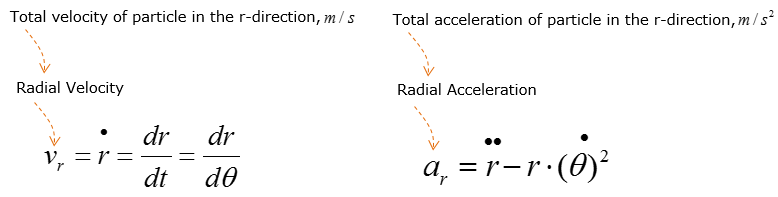Putting a little bit of details to the radial acceleration equation, the equation can be described as follows.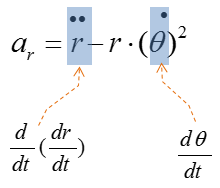Now let's take look at the equation describing the angular movement (movement in theta direction)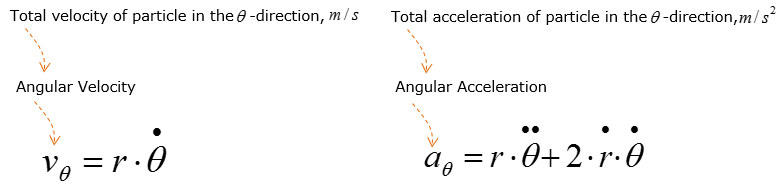Putting a little bit of details to the radial acceleration equation, the equation can be described as follows.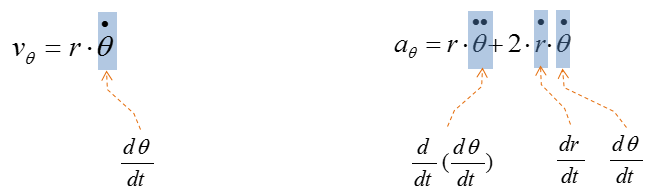Example 01 >   A slotted link is pinned at O, and as a result of the constant angular velocity = 3 rad/s it drives the peg P for short distance along the spiral guide r = 0.4 theta (m), where theta is in radians.  Determine the velocity and acceleration of the particle at the instant it leaves the slot in the link, ie. When r = 0.5m.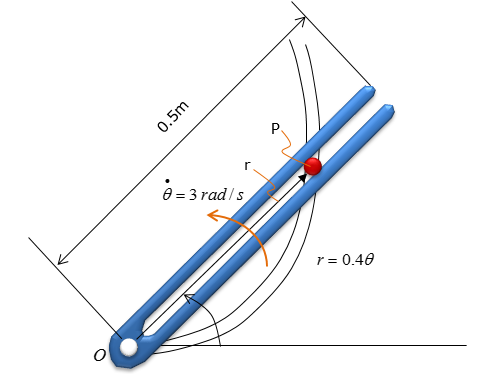Step 1 > Try to get an intuitive understanding on the movement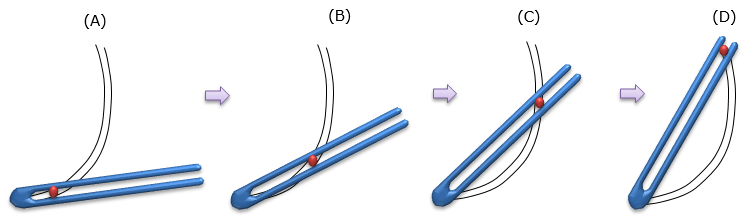Step 2 > Set the reference coordinate system and directions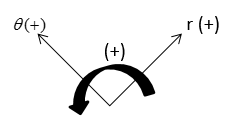Step 3 > Indicate all the vectors given to you and the vectors that you have to figure out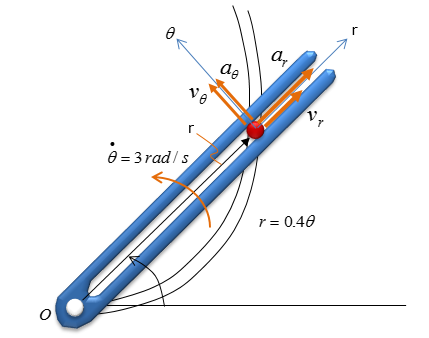Step 4 > Take derivatives of the position function r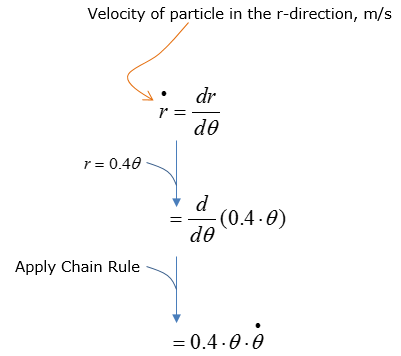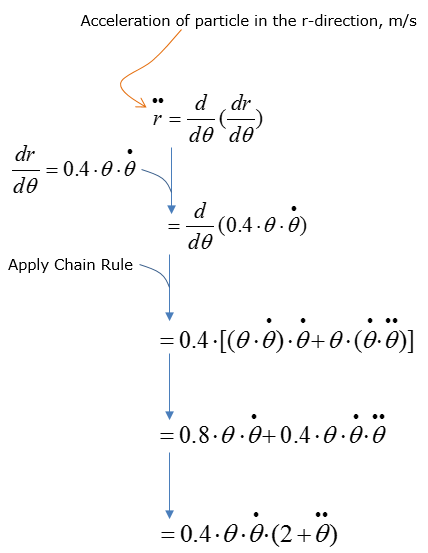Step 5 > Calculate velocity and acceleration in r-direction   Utilize the velocity and acceleration expressions in the r-directions from the 1st step to calculate the total velocity and acceleration in the r-direction. Consider the velocity.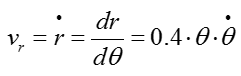Before solving for Vr, the angular displacement (theta) must be known. Using the position equation given,  theta can be computed since the displacement of the particle is given as 0.5 m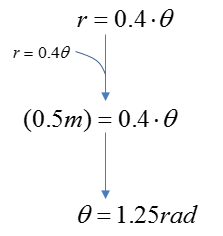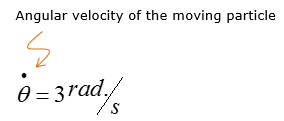Since both the angular displacement and velocity are now known quantities, they can be used to calculate for Vr.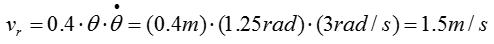Now Consider the acceleration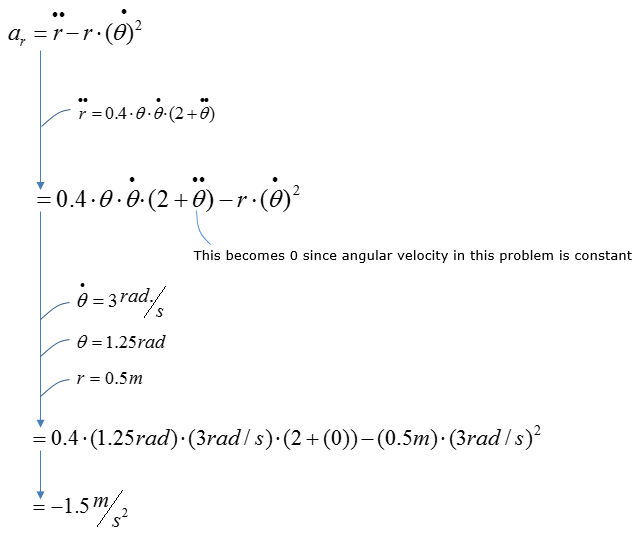Step 6 > Calculate velocity and acceleration in theta-direction   Utilize the velocity and acceleration expressions in the r-directions from the 1st step to calculate the total velocity and acceleration in the theta-direction.   Consider the velocity.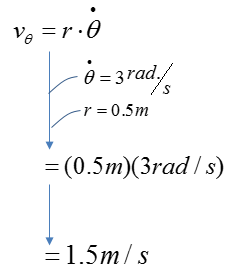Now Consider the acceleration.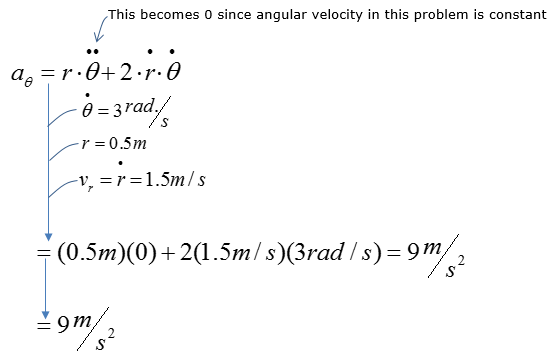Step 7 > Calculate the total velocity and acceleration of the moving particle   Let's consider the acceleration first. I suggest you to draw the vectors on the problem set using the values that were obtained in previous steps as below.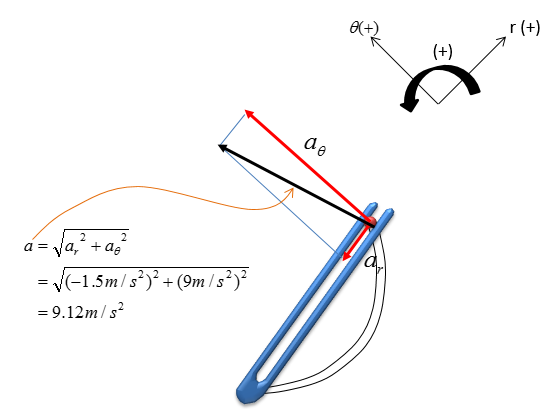Now let's consider the velocity part. I suggest you to draw the vectors on the problem set using the values that were obtained in previous steps as below.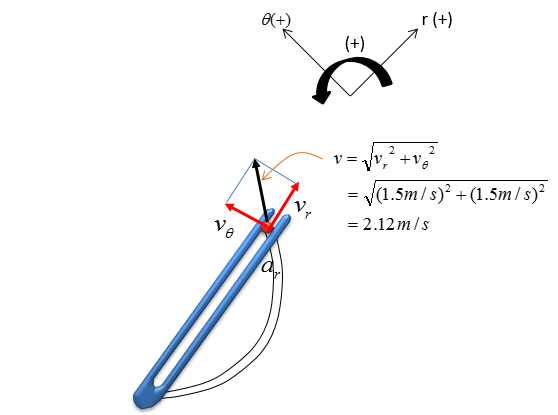Reference :    Scotch yoke Mechanism (YouTube)